# 关于二分查找算法

## 1 传统二分查找

### 1.1 算法描述

• (1) 如果中间值偏小，说明中间值及其左边的值都比目标值小，全部剔除出列表，继续下 一轮迭代；
• (2) 如果中间值偏大，说明中间值及其右边的值都比目标值大，全部剔除出列表，继续下 一轮迭代；
• (3) 如果中间值与目标值相等，则已经找到，中间点就是目标值的位置。
• (4) 如果列表为空，说明整个列表中都没有与目标值相等的元素。

/* search T in array A witch contain n elements, and return index of T.
* Return -1 if T not exists in A. */
int binary_search(int *A, int n, int T) {
int L = 0;
int R = n - 1;
int m = -1;
while (L <= R) {
m =  (L + R) / 2;
if (A[m] < T)
L = m + 1;
else if (A[m] > T)
R = m - 1;
else
return m;
}
return -1;
}


• 第一轮迭代，列表为 {1,2,3,5,7,8,9,9}，中间值是5，比7小，剔除5及左边所有元素；
• 第二轮迭代，列表为 {7,8,9,9}，中间值是8，比7大，剔除8及右边所有元素；
• 第三轮迭代，列表为 {7}，中间值是7，找到结果，算法结束。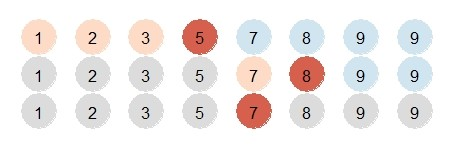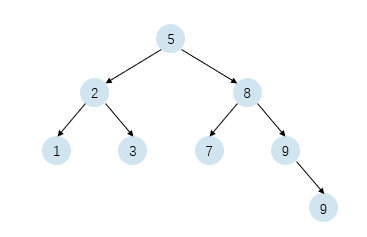1 每次迭代，要么使算法结束，要么列表中减少至少一个元素
2 每次缩短列表，不能删除任何目标值
3 找到目标值或者列表为空时结束

### 1.2 过早退出的错误

int binary_search(int *A, int n, int T) {
int L = 0;
int R = n - 1;
int m = -1;
while (L < R) { // 应该是 L <= R
m =  (L + R) / 2;
if (A[m] < T)
L = m + 1;
else if (A[m] > T)
R = m - 1;
else
return m;
}
return -1;
}


• 第一轮迭代，列表为 {1,2,3,5,7,8,9,9}，中间值是5，比7小，剔除5及左边所有元素；
• 第二轮迭代，列表为 {7,8,9,9}，中间值是8，比7大，剔除8及右边所有元素；
• 第三轮迭代，列表为 {7}，循环退出，返回-1。

### 1.3 无法退出的错误

int binary_search(int *A, int n, int T) {
int L = 0;
int R = n - 1;
int m = -1;
while (L <= R) {
m =  (L + R) / 2;
if (A[m] < T)
L = m; // 应该为 L=m-1
else if (A[m] > T)
R = m - 1;
else
return m;
}
return -1;
}


• 第一轮迭代，列表为 {1,2,3,5,7,8,9,9}，中间值是5，比4大，剔除5右边所有边所有元素；
• 第二轮迭代，列表为 {1,2,3}，中间值是2，比4小，剔除2左边所有元素，不剔除2；
• 第三轮迭代，列表为 {2,3}，中间值是2，比4小，剔除2左边所有元素，没有剔除2；
• 第四轮迭代，列表为 {2,3}，中间值是2，比4小，剔除2左边所有元素，没有剔除2；
• 第n轮迭代，列表为 {2,3}，中间值是2，比4小，剔除2左边所有元素，没有剔除2；

int binary_search(int *A, int n, int T) {
int L = 0;
int R = n - 1;
int m = -1;
while (L <= R) {
m =  (L + R) / 2;
if (A[m] < T)
L = m - 1;
else if (A[m] > T)
R = m; // 应该为 R = m - 1;
else
return m;
}
return -1;
}


### 1.4 剔除目标值的错误

int binary_search(int *A, int n, int T) {
int L = 0;
int R = n - 1;
int m = -1;
while (L <= R) {
m =  (L + R) / 2;
if (A[m] <= T) // 应该为 if (A[m] < T)
L = m + 1;
else if (A[m] > T)
R = m - 1;
else
return m;
}
return -1;
}


else if (A[m] > T)


else if (A[m] >= T)


### 1.5 整型溢出的错误

// 加法有整型溢出风险
m = (L + R) / 2;

// 第一种修改方法
// 使用符号位保存溢出的比特位，在除以2之后符号位一定会是0。
// 这种写法使用一次加法，一次除法
m = (int)(((unsigned int)L + (unsigned int)R) / 2);

// 第二种修改方法
// 利用恒等式 (L+R)/2 = L+(R-L)/2
// 将可能溢出的加法变换为不会溢出的加法。不会溢出是因为
// L+(R-L)/2 < R
// 这种写法使用一次加法，一次减法，一次除法
m = L + (R - L) / 2；

// 有的人会将 a/2 替换为 (a >> 1)
// 常用于右移指令比除法快的架构中，常见架构都是如此。
// 要注意 >> 运算符优先级低于 +-*/ 四则运算，需要括号。
m = L + ((R - L) >> 1);


## 2 中途不退出二分查找

/* search T in array A witch contain n elements, and return index of T.
* Return -1 if T not exists in A. */
int binary_search_less_compare(int *A, int n, int T) {
int L = 0;
int R = n - 1;
int m = -1;

if (n <= 0)
return -1;

while (L < R) {
m = (L + R + 1) / 2;
if (A[m] > T)
R = m - 1;
else
L = m;
}

if (A[L] == T)
return L;
return -1;
}


• 第一轮迭代，列表为 {1,2,3,5,7,8,9,9}，中间值是7，不比目标值7小，剔除7左边的元 素；
• 第二轮迭代，列表为 {7,8,9,9}，中间值是9，比7大，剔除9及右边所有元素；
• 第三轮迭代，列表为 {7,8}，中间值是8，比7大，剔除8以及右边所有元素；
• 最后列表为 {7}，仅剩一个元素，循环退出，判断中间值与目标值相等，列表地址，也就 是7的地址，算法退出。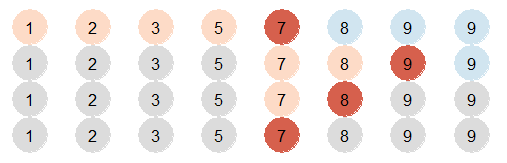1. 中途不退出二分查找使用中间或中间偏右的元素为中心节点，而传统二分查找以中间或 中间偏左的元素为中心节点。
2. 中途不退出二分查找在仅剩一个元素时退出迭代，而传统二分查找在中间元素与目标值 相等或者列表为空时退出。
3. 中途不退出二分查找在迭代中减少了一轮比较。
4. 中途不退出二分查找使用 L=m 而不是 L=m-1。
5. 中途不退出二分查找在循环结束时需要一次比较。
6. 为了防止未定义行为，上述程序在开始处添加了列表为空的判断。

1 每次迭代，列表中减少至少一个元素
2 每次缩短列表，不能删除任何目标值
3 列表至少包含一个元素

### 2.1 晚退出的错误

int binary_search_less_compare(int *A, int n, int T) {
int L = 0;
int R = n - 1;
int m = -1;

if (n <= 0)
return -1;

while (L <= R) { // 应该是 L<R
m =  (L + R + 1) / 2;
if (A[m] > T)
R = m - 1;
else
L = m;
}

if (A[L] == T)
return L;
return -1;
}


### 2.2 中间偏左的错误

int binary_search_less_compare(int *A, int n, int T) {
int L = 0;
int R = n - 1;
int m = -1;

if (n <= 0)
return -1;

while (L < R) {
m = (L + R) / 2; // 应该为 m = (L + R + 1) / 2;
if (A[m] > T)
R = m - 1;
else
L = m;
}

if (A[L] == T)
return L;
return -1;
}


int binary_search_less_compare(int *A, int n, int T) {
int L = 0;
int R = n - 1;
int m = -1;

if (n <= 0)
return -1;

while (L < R) {
m = (L + R) / 2;
// 因为m是中间偏左，为了保证至少剔除一个元素，
// 要么至少 L=m+1，要么 R=m。
if (A[m] < T)
L = m + 1;
else
R = m;
}

if (A[L] == T)
return L;
return -1;
}


### 2.3 没有剔除元素的错误

int binary_search_less_compare(int *A, int n, int T) {
int L = 0;
int R = n - 1;
int m = -1;

if (n <= 0)
return -1;

while (L < R) {
m = (L + R + 1) / 2;
if (A[m] > T)
R = m; // 应该是 R = m - 1;
else
L = m;
}

if (A[L] == T)
return L;
return -1;
}


### 2.4 剔除了目标值的错误

int binary_search_less_compare(int *A, int n, int T) {
int L = 0;
int R = n - 1;
int m = -1;

if (n <= 0)
return -1;

while (L < R) {
m = (L + R + 1) / 2;
if (A[m] > T)
R = m - 1;
else
L = m + 1; // 应该是 L = m;
}

if (A[L] == T)
return L;
return -1;
}


## 3 upper bound

### 3.1 算法描述

upper bound 是最常用的，它返回的是列表中第一个比目标值大的元素，也就是第一个插入 位置。 返回值总是目标值的插入位置，如果插入位置有值，则将该位置以及右边所有元素向右平移 一次，再插入。

 地址 0 1 2 值 1 1 3

1. 如果中间值等于目标值，可以将中间值及其左边的元素都剔除；
2. 如果中间值大于目标值，也可以将中间值及其左边的元素都剔除；
3. 如果中间值小于目标值，可以将中间值右边的元素剔除，不剔除中间值。

int bs_upper_bound(int *A, int n, int T) {
int L = 0;
int R = n;
int m = -1;
while (L < R) {
m = (L + R) / 2;
if (T >= A[m]) {
L = m + 1;
} else {
R = m;
}
}
return L;
}


• 第一轮迭代，列表为 {1,2,3,5,7,8,9,9,∞}，中间值是7，比目标值7小，剔除7左边的元 素；
• 第二轮迭代，列表为 {7,8,9,9,∞}，中间值是9，剔除9及左边所有元素；
• 第三轮迭代，列表为 {9,∞}，中间值是9，剔除9；
• 最后列表为 {∞}，中间值是∞，比9大，将其剔除，列表为空，算法结束，此时当前列表 的地址为列表后紧跟着的节点，将其返回。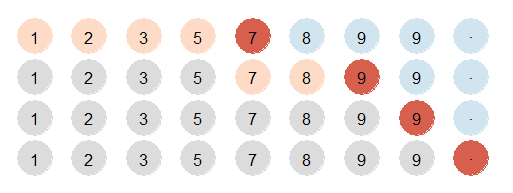1 每次迭代，列表中减少至少一个元素
2 除最后一轮迭代外，不能将目标值右边的元素剔除
3 列表为空时，算法结束
4 不能对n寻址，因为没有值

### 3.2 无法剔除元素的错误

int bs_upper_bound(int *A, int n, int T) {
int L = 0;
int R = n;
int m = -1;
while (L < R) {
m = (L + R) / 2;
if (T >= A[m]) {
L = m; // 应为 L = m + 1;
} else {
R = m;
}
}
return L;
}


### 3.3 剔除了目标值后面元素的错误

int bs_upper_bound(int *A, int n, int T) {
int L = 0;
Int R = n;
int m = -1;
while (L < R) {
m = (L + R) / 2;
if (T >= A[m]) {
L = m + 1;
} else {
R = m - 1; // 应外 R = m;
}
}
return L;
}


### 3.4 初始化错误

int bs_upper_bound(int *A, int n, int T) {
int L = 0;
int R = n - 1; // 应为 int R = n;
int m = -1;
while (L < R) {
m = (L + R) / 2;
if (T >= A[m]) {
L = m + 1;
} else {
R = m;
}
}
return L;
}


### 3.5 剔除条件错误

int bs_upper_bound(int *A, int n, int T) {
int L = 0;
int R = n;
int m = -1;
while (L < R) {
m = (L + R) / 2;
if (T > A[m]) {
L = m + 1;
} else {
R = m;
}
}
return L;
}


if (T >= A[m]) 写成了 if (T > A[m])，这令 T=A[m]的情况下不能剔除任何节点，违反 断言1。

## 4 lower-bound

lower bound 算法与 upper bound 类似，它返回的结果是列表中第一个等于目标值的元素 的地址，如果列表中没有目标值，那么它将返回大于目标值的第一个元素。

 地址 0 1 2 值 1 1 3

upper bound 和 lower bound 有一个附带的性质： upper bound - lower bound = 目标值的元素的个数。

int bs_lower_bound(int *A, int n, int T) {
int L = 0;
int R = n;
int m = -1;
while (L < R) {
m = (L + R) / 2;
if (T <= A[m]) {
R = m;
} else {
L = m + 1;
}
}
return L;
}


1 每次迭代，列表中减少至少一个元素
2 除最后一轮迭代外，如果列表中没有目标值，那么大于目标值的第一个节点不能剔除
3 列表为空时，算法结束
4 不能对n寻址，因为没有值
5 如果列表中有目标值，最左边的目标值不能剔除

## 5 Knuth 的二分查找

Uniform Binary search 在查找之前，提前生成了一个表，用于高效的计算中间值。表中的数据如下：

\begin{align*} delta_0 &= \frac{n+1}{2} \\ delta_1 &= \frac{n+2}{4} \\ delta_3 &= \frac{n+4}{8} \\ delta_4 &= \frac{n+8}{16}\\ \cdots & \end{align*}

#define N 10
#define LOG_N 4
static int delta[LOG_N];

void make_delta(int N) {
int power = 1;
int i = 0;
do {
int half = power;
power <<= 1;
delta[i] = (N + half) / power;
} while (delta[i++] != 0);
}

int unisearch(int *A, int T) {
int i = delta-1;  /* midpoint of array */
int d = 0;

while (1) {
if (T == A[i]) {
return i;
} else if (delta[d] == 0) {
return -1;
} else {
if (T < A[i]) {
i -= delta[++d];
} else {
i += delta[++d];
}
}
}
}

int main(void) {
int A[N] = {1,3,5,6,7,9,14,15,17,19};
make_delta(N);
unisearch(A, 15);
return 0;
}


## 6 指数查找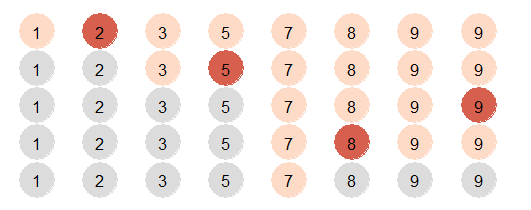## 7 比例二分查找

m = (T-A[L])/(A[R]-A[L]) * n;